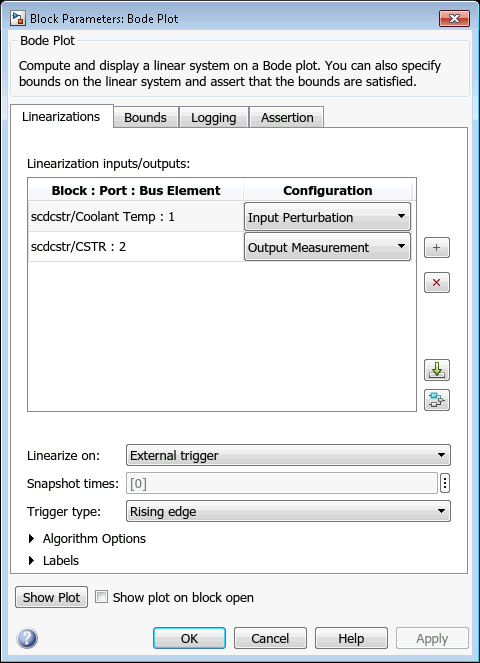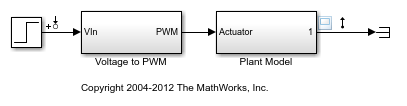# setlinio

Save linear analysis points to Simulink model, Linear Analysis Plots block, or Model Verification block

## Syntax

``setlinio(mdl,io)``
``setlinio(blockpath,io)``
``oldio = setlinio(___)``

## Description

example

````setlinio(mdl,io)` writes the analysis points specified in `io` to the Simulink® model `mdl`.```

example

````setlinio(blockpath,io)` sets the specified analysis points to the specified Linear Analysis Plots block or Model Verification block.```

example

````oldio = setlinio(___)` returns the current set of analysis points in the model or block and replaces them with `io` using any of the previous syntaxes.```

## Examples

collapse all

```model = 'magball'; open_system(model) ```Create a vector of analysis points for linearizing the plant model:

• Input perturbation at the output of the Controller block

• Open-loop output at the output of the Magnetic Ball Plant block

```io(1) = linio('magball/Controller',1,'input'); io(2) = linio('magball/Magnetic Ball Plant',1,'openoutput'); ```

Write the analysis points to the `magball` model.

```setlinio(model,io); ```

The analysis points in `io` are added to the model as annotations. You can then save the model to store the analysis points with the model.

```open_system('scdcstr') ```Create analysis points for finding the transfer function between the coolant temperature and the residual concentration.

• Input perturbation at the output of the Coolant Temp block

• Output measurement at the CA output of the CSTR block

```io(1) = linio('scdcstr/Coolant Temp',1,'input'); io(2) = linio('scdcstr/CSTR',2,'output'); ```

Set the analysis points in the Bode Plot block.

```setlinio('scdcstr/Bode Plot',io); ```

View the analysis points in the Bode Plot Block Parameters dialog box.

```open_system('scdcstr/Bode Plot') ```During simulation, the software linearizes the model using the specified analysis, and plots the magnitude and phase responses for the resulting linear system.

```mdl = 'scdpwm'; open_system(mdl) ```This model is configured with analysis points for finding the combined transfer function of the PWM and plant blocks.

Create analysis points for finding the transfer function of just the plant model.

```io(1) = linio('scdpwm/Voltage to PWM',1,'input'); io(2) = linio('scdpwm/Plant Model',1,'output'); ```

Store the analysis points to the model, and save the previous analysis point configuration.

```oldio = setlinio(mdl,io) ```
```2x1 vector of Linearization IOs: -------------------------- 1. Linearization input perturbation located at the following signal: - Block: scdpwm/Step - Port: 1 2. Linearization output measurement located at the following signal: - Block: scdpwm/Plant Model - Port: 1 ```

## Input Arguments

collapse all

Simulink model name, specified as a character vector or string. The model must be in the current working folder or on the MATLAB® path.

If the model is not open or loaded into memory, `setlinio` loads the model into memory.

Analysis point set, specified as a linearization I/O object or a vector of linearization I/O objects.

Each linearization I/O object has the following properties:

PropertyDescription
`Active`

Flag indicating whether to use the analysis point for linearization, specified as one of the following:

• `'on'` — Use the analysis point for linearization. This value is the default option.

• `'off'` — Do not use the analysis point for linearization. Use this option if you have an existing set of analysis points and you want to linearize a model with a subset of these points.

`Block`

Full block path of the block with which the analysis point is associated, specified as a character vector.

`PortNumber`

Output port with which the analysis point is associated, specified as an integer.

`Type`

Analysis point type, specified as one of the following:

• `'input'` — Input perturbation

• `'output'` — Output measurement

• `'loopbreak'` — Loop break

• `'openinput'` — Open-loop input

• `'openoutput'` — Open-loop output

• `'looptransfer'` — Loop transfer

• `'sensitivity'` — Sensitivity

• `'compsensitivity'` — Complementary sensitivity

For more information on analysis point types, see Specify Portion of Model to Linearize.

`BusElement`

Bus element name with which the analysis point is associated, specified as a character vector or `''` if the analysis point is not a bus element.

`Description`

User-specified description of the analysis point, which you can set for convenience, specified as a character vector.

Linear Analysis Plots block or Model Verification block, specified as a character vector or string that contains its full block path. The model that contains the block must be in the current working folder or on the MATLAB path.

## Output Arguments

collapse all

Old analysis point set, returned as a linearization I/O object or a vector of linearization I/O objects.